# Tail

What’s algorithm: it is formally a finite sequence of steps that lead to execution of a task How can cite examples of algorithms the algorithms of basic operations (addition, multiplication, division and subtraction) of real numbers decimals.
An algorithm has four important characteristics: Finite; Definition; Entries; outputs.

Forms of representation: narrative description; Flowchart conventional pseudocode.
Basic control structures: Sequence simple; Alternatives (conditional) Repeat (allows to repeat the actions)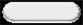-> Terminal: indicates the point where it starts or stops the flow of a program.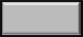Processing indicates the arithmetic or calculation of a formula, etc. distributions of values.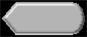Display: For information shown in the video.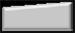Keyboard: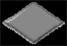Decision: indicates verification or comparison in the form of a question, and there may be one answer: yes or no.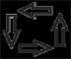Arrows: indicate the direction.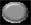Connector:
used when you need to partition the diagram.

Storing data in memory: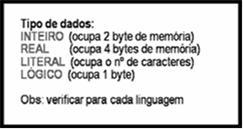• Each memory cell occupies one byte.
• Each data type requires a certain amount of memory to store information
• To retrieve a specific information in memory would be necessary to know the data type of this information (ie, the number of bytes it occupies
in memory) and starting position in this set of bytes in memory. For example, to retrieve information “banana” We know that this is 6 bytes and starts at position 18.
To circumvent this problem was created the concept of variable. A variable contains a reference to the original address and it is known that the number of bytes in memory occupied by its data type.

Variables and data types (basic): All variables belong to a data type that defines a set of values that it can receive (store).

Data Type: Number: integer and real, literal or character; Logic

To develop an algorithm we must first divide the problem into three key stages: Input, Processing and Saida.

Among these forms of representation of the best known algorithms are: Narrative Description; conventional flowchart, Pseudocode, also known as Language or Structured Portugol.

<nome_do_algoritmo> Algorithm: Algorithm is a word that indicates the beginning of the definition of an algorithm in the form of pseudocode.

<nome_do_algoritmo>: is a symbolic name given to the algorithm in order to distinguish them from others.
<declaração_de_variáveis>: option consists of a portion where they are declared the global variables used in the main algorithm, and possibly in subalgoritmos.
<subalgoritmos>: consists of an optional portion of the pseudocode where they are defined subalgoritmos.

Start:

is the word that delimit the beginning of the instruction set of the body of the algorithm.

<corpo of algoritmo>: Are the instructions (commands, …)
FimAlgoritmoi: is the word that delimit the end of the instruction set of the body of the algorithm.

Integer types: integer types are characterized as the positive or negative figures. Excluding any of these fractional number. Examples of such data has the values: 35, 0, -56, 1024 among others;
Real types: they are characterized as real types, the figures positive and negative and fractional numbers. Examples of such data has the values: 35, 0, -56, 1.2, -45,987 among others;
Character types: types are characterized as characters, the sequences of letters, numbers and special symbols. A sequence of characters must be indicated by quotation marks (). This data type is also known as alpha-numeric, string, literal
or chain. Example: Software, Alpha Street, 52 Apt 1, Phone 574-9988, 04387-030,, 7 others;
Logical types: logical types are characterized as data with value true and false.

Create rules for the names (identifiers) of variables \ constant
The variables and constants have a name (label) that identify them within the code \ pseudocode. This name must be created according to the following principles:
a) The first character must ALWAYS be a letter;
b) The names should be composed of characters belonging to the letters of the alphabet and numbers. If the handle is more than one word, we can separate them with underline
c) There can be no blank spaces between the characters of the identified
d) The names chosen should be explanatory of its contents;
e) Variable names longer inhibit encoding (check size depending on the programming language that is);
f) Do not use accents or cedilla;
g) The names given to variables can not be the same names as reserved words of the language being used.

Arithmetic operators: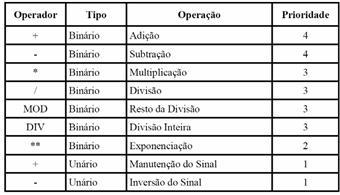Logical Operators: The logical or Boolean operators are used to combine relational expressions. Also return as a result of logical values true or false.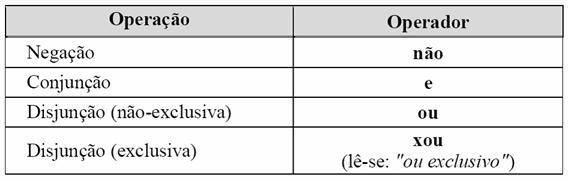Truth Table: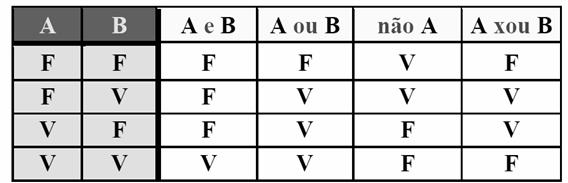Instructions primitive: they are the basic commands that perform tasks critical to the operation of computers, as input and output data (communication with the server and peripheral devices), and transferring these into memory. These types of instruction are present in most programming languages.

Command Assignment: The assignment statement or simply assignment, is the primary way of storing information in a variable. The following is an example of an algorithm using the assignment statement:
Algorithm exemploAtribuição
Var
preço_unit, preço_tot: real
quant: integer
Initiation
preço_unit: = 5.0
quant: = 10
preço_tot: * quant = preço_unit
FimAlgoritmo.
Note:
visualg in the assignment statement is: = (has to be changed to <-)

Output Command Data (WRITE): the commands output data are the means by which information in the computer memory are placed in the output devices so that users can view them. The following is an example of an algorithm using the command’s output:
Var preço_unit, preço_tot: real
quant: integer
Initiation
preco_unit: = 5.0
quant: = 10
preço_tot: * quant = preço_unit
Type (preço_tot)
End.
Note:
visualg in the assignment statement is: = (has to be changed to <-)
The commands input data (READ) is the means by which user information is transferred to computer memory, so they can be used in programs. The following is an example of an algorithm using the command input data:
Var preço_unit, preço_tot: real
quant: integer
Initiation
quant)
preço_tot: * quant = preço_unit
Write (preço_tot)
Fimalgoritmo
Note:
visualg in the assignment statement is: = (has to be changed to <-)

STRUCTURES OF CONTROL FLOW OF EXECUTION: sequential structures, decision structures, repetition structures.

Sequential structure: In the sequential structure of the commands of an algorithm are executed in a predetermined sequence. Each command is executed only after the end of the previous command.

Decision Structures: In this type of structure the flow of instructions to be followed is chosen depending on the outcome of the evaluation of one or more conditions. A condition is a logical expression. The classification of the structures of decision is made according to the number of conditions that must be tested to decide which path to follow. Under this classification, have three types of decision-making structures:

Simple Structure of Decision (If … Then) Structure Decision Made (if … then … else)

Structure Decision Type Multiple Choice (Choose … If … Else)

Decision Structures Multiple Type Case (Case fim_caso … but …): This type of structure is a generalization of the construction if, where only one condition was assessed and two paths could be followed. In the structure of decision as if there may be one or more conditions to be tested and a different command associated with each of these.

What are the steps for building an algorithm: Answer: The main ones are: Understanding the problem, identify the inputs, ie, identify the data to be provided to enable solving the problem, identify the outputs that should be generated as a result of the solution; Determine what must be done to transform inputs into outputs (processing). Construct the algorithm, using a form of representation.

What is logic programming? Answer: Logic programming is nothing more than a way to write a program, or is related to the order in which we organize the program instructions.

Data type: is related to the type of information we want to store in computer memory (eg if an integer is a number with decimals (real), character or logical).
Variables
: When we refer to the variable, in terms of computer programming, we are dealing with a region of memory (the computer) previously identified whose purpose is to store data or information from a program for a certain period of time. Computer memory is organized such that a cabinet with several divisions. Each division being identified by an address. The computer stores the data in these divisions, and in each division can only store a given, and every time the computer store in a given one of these divisions, the data that was previously stored is cleared. The content may change, but only for a given time can be stored in that
division. The computer identifies each division through an address in hexadecimal format, and programming languages allow the address or name each memory location, making reference to a memory address. A variable consists of two basic elements: the content, the value of the variable identifier, a name given to the variable to enable its use.

Analyze the algorithms below and tell me what is printed on the screen to be performed:
algorithm teste01
var
A, B: integer
inicio
A <-10
B <- 20
Escreval (B)
B <- 5
Escreval (A, B)
Fimalgoritmo
Write B / / value printed: 20
Escrver A, B / / values printed: 10:05

algorithm teste02
var
A, B, C: integer
inicio
A <- 30
B <- 20
C <- A + B
Escreval (C)
B <- 10
Escreval (B, C)
C <- A + B
Escreval (A, B, C)
Fimalgoritmo
50 / / print value of C
10 50 / / print the values B and C
40 October 30 / / prints the values A, B, C

algorithm teste03 ”
var
X: integer
Z, Y: real
inicio
X <- 1
Y <- 2
Z <- 2 * YX
Escreval (Z)
X <- 5
Second / / print value of Z
5 6 2 / / print values of X, Y, Z

1 - A bank will grant a special credit to their customers, variable with the average balance in
last year. Make an algorithm that reads the average balance of a customer and calculate the value of
credit according to the chart below. Show a message informing the average balance and
value of the claim.
Average Balance / Percent
0-200 / no credit
201-400 / 20% of the value of the average balance
401-600 / 30% of the value of the average balance
over 601 / 40% of the average balance
Algorithm SaldoMédio
Var
Salmedio, Vlrcred: real
Name: character

Home
Escreval (“Enter the customer’s name:”)
Escreval (“Enter the average balance:”)
If Salmedio <= 200 then
Vlrcred <- 0
Senao
If Salmedio <= 400 then
Vlrcred <- (Salmedio * 20) / 100
Senao
If Salmedio <= 600 then
Vlrcred <- (Salmedio * 30) / 100
Senao
Vlrcred <- (Salmedio * 40) / 100th
ENDIF
ENDIF
ENDIF
Escreval (“Customer Name”, Name)
Escreval (“Average Balance”, Salmedio)
Escreval (“Value of Credit:” Vlrcred)
Fimalgoritmo
Algorithm to repeat at the end, keep asking:

algorithm MediaAluno ”

/ / Function: Calculate the arithmetic average of 30 students
/ Author: de Freitas Veronice
/ / Date: 12/03/2010
/ / Declarations section

var
NOTE 1, NOTE2 MEDIA REAL
CONT: integer
inicio
CONT <- 0
repeat
MEDIA <- (NOTE 1 NOTE2 +) / 2
escreval (“Average =”, MEDIA)

CONT <- + 1 CONT
until CONT = 30 / / to test students with less change the value 30 (eg 5)

fimalgoritmo

1 – Write the pseudocode equivalent to the flowchart below.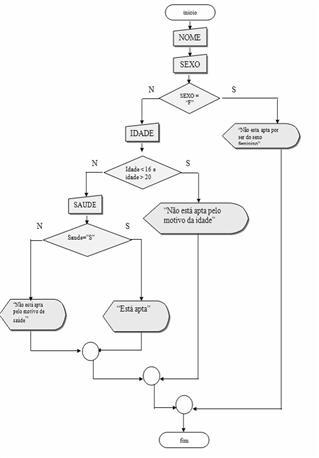Algorithm Enlistment
Var
behalf, health, sex: character
age:
all
Home

Write (Enter sex F / M:)
If Sex = F
then
Escreval (Does not fit to be female)
Senao
Escreval (Enter age:)
If (age <= 16) and (age> = 20) then
Escreval (Does not fit by reason of age)
Senao
Escreval (Health Among suitable for S / N:)
If S = then health
Escreval (This fit)
Senao
Escreval (Not suitable for this reason health)
ENDIF
ENDIF
ENDIF

Fimalgoritmo

1 - What is the difference of structures of repetition: (1) REPEAT / UNTIL (2)WHILE / END WHILE (3) FOR / END FOR

Answer: a) REPEAT / UNTIL: Executes a block of statement until the condition is TRUE (the block of instruction is executed at least one time) “the condition is tested after the instructions.
Example:
Repeat
<instruções>.
Until RESP = S
2) WHILE / END WHILE:
Executes a block of statement until the condition is FASA
Example: (if the variable is RESP = N will not be executed at all.
AS RESP = S KNIFE
<instruções>
END WHILE
3) FOR / END FOR:
Executes a block of instruction a specified number of times.
Example: In this case the number of times the instructions are executed is determined by the initial value and final value.
FOR <variable> of <value inicial> until <value final> knife
<instruções>
fimpara

VECTORS: San homogeneous data structures that allow us to work with multiple information in one variable; The information stored in a vector must necessarily be the same type, which is why, the vectors are called homogeneous data structures, or an array of dimension.

Example:

Var
vet: array [1 .. 5] of integer
Home
vet  <- 1522
fimalgoritmo

1 – Set vectors and matrices. Answer: Data structures allow homogeneous grouping various information within a single variable. This grouping occurs always obeying the same type of data, and for this reason that these structures are called homogeneous.
2 – How are known vectors and matrices?:

Answer: Indexed variables, composite variables, arrays, vectors, matrices, tables or arrays in memory
3 – How is the declaration of the two vectors to store products 20 and 20 prices?

PRODUCT: array [1 .. 20] of character
PRICE: array [1 .. 20] of real

1 - Perform the calculation of the amount of gallons of fuel spent on a trip, using a car which is 12 KM per liter. To obtain the calculation, the user must provide the time spent and average speed during the trip. Thus, you can get the distance with the formula DISTANCE = SPEED * TIME. Having the distance value, just calculate the amount of gallons of fuel used in traveling to the formula: = LITROS_GASTOS DITANCIA / 12. The program should display the values of average speed, time spent on the trip, distance traveled, number of gallons used during the trip.
Note:

Input data: TIME and SPEED
Processing: calculate distance using the formula of the utterance and then calculate Litres expenses (see the formula in the utterance)

/ / Function: Calculate the distance and expense
/ / Author: Joao Neto
/ / Date: 01/06/2010
/ / Declarations section
var
inicio
/ / Data Entry
escreval (“Enter the average speed)
escreval (“Enter the time spent)
/ / Processing
litros_gastos <- distance / 12
/ / Output
escreval (“The distance was” distant, “km”, “and the liters spending was” litros_gastos, “L”)
fimalgoritmo

2 - In a parts factory, base pay of a worker in the manufacturing line is R \$ 240.00. In addition to base salary, the worker takes an additional productivity based on quantity of pieces he makes a month that is paid at the discretion (note: use an additional variable to make the calculations): If the number of parts is less, or , 500: only the base salary Example: ADDITIONAL <- 0

If the number of parts is greater than 500 and less, or 750: \$ 0.50 per piece made up of 500
Example: ADDITIONAL <- (QTDE 500) * 12:50
If the number of parts is greater than 750: get \$ 350.00 (fixed) plus \$ 0.75 per piece made up of 750.
Example: ADDITIONAL <- (QTDE 750) * 0.75 + 350
Develop an algorithm that allows to enter the number of parts manufactured by a factory worker and print your salary will be calculated.
Example: SALARIO_FINAL <- 240 + ADDITIONAL

/ / Function: Calculating Wage Gain in Month
/ / Author: Joao Neto
/ / Date: 01/06/2010
/ / Declarations Section
var

inicio
/ / Assigning values
salario_base <- 240.00

/ / Data Entry
escreval (“Enter Number of Parts Made in the Month”)

escreval (“The final value is \$” salario_final)
Senao
escreval (“The final value is \$” salario_final)
Senao
escreval (“The final value is \$” salario_final)
ENDIF
ENDIF
ENDIF
fimalgoritmo

3 - Following the release of government for the tuition of health plans, people began to do research to find a good plan, not too expensive. A salesman for a health plan presented in the following table. Create a program to enter the name and age of a person and show the name and value that it must pay (using conditional structure chained).
Note:
Input data: Name and Age
Processing: Calculate the value
Output: NAME and VALUE
Until 10 years \$ 30.00
Above 10 to 29 years \$ 60.00
Above 29 to 45 years \$ 120.00
Above 45 to 59 years \$ 150.00
Above 59 to 65 years \$ 250.00
Over 65 years \$ 400.00.

algorithm “Health Insurance”
/ / Function: Compute the value according to age
/ / Author: Joao Neto
/ / Date: 02/06/2010
/ / Declarations Section
var
Name: character
age: all
value: real

inicio
/ / Data Entry
escreval (“Enter the customer name”)
escreval (“Enter age”)

If (age <= 10) then
value <- 30.00
escreval (“The amount payable is R \$”, value)
Senao
If (age> = 10) and (age <= 29) then
value <- 60.00
escreval (“The value to be paid is \$”, value)
Senao
If (age> 29) and (age <= 45) then
value <- 120.00
escreval (“The amount payable is R \$”, value)
Senao
If (age> 45) and (age <= 59) then
value <- 150.00
escreval (“The amount payable is R \$”, value)
Senao
If (age> 59) and (age <65) then
value <- 250.00
escreval (“The amount payable is R \$”, value)
Senao
if (age> 65) then
value <- 400.00
escreval (“The amount payable is R \$”, value)
ENDIF
ENDIF
ENDIF
ENDIF
ENDIF
ENDIF

fimalgoritmo

5 - A company did a survey to find out if people liked it or not one of their new products launched in the market. Collected for this: the sex of the interviewee (M or F), its age and its response (Y or N). Given that 1,000 people were interviewed, to develop an algorithm to compute and report (Note: declare six variables eg CONT1, CONT2, CONT3, CONT4, CONT5, CONT6 to check all items below, do not forget the repetition structure for make all entries and also initialize the
counter variables before the structure of repetition):
a) Number of people who responded yes;
b) Number of people who answered No;
c) How many people greater than or equal to 18 years liked
product;
d) How many people under 18 did not like the product;
e) How many people greater than or equal to 18 years, female
disliked the product;
f) How many people under 18 years, male
liked the product.
Observation
Processing: TAKE CONTROL FOR ALL ACCOUNTANTS
(The CONT1 CONT6)
Output: Print the answer to all survey items (alternative
to) f))
Answer: algorithm “Survey on our product”
/ / Function: Calculate the quantity, old people, and evaluation of our product
/ / Author: Joao Neto
/ / Date: 02/06/2010
/ / Declarations section
var
cont1, cont2, cont3, cont4, cont5, cont6, age: integer
response, sex, continues: character
inicio
/ / Section Command
still <- ‘S’
while continuing = “S” knife
escreval (Search to see if you liked our product. “)
write (“Enter Gender F for female or M for male”)
while (sex <> “F”) and (sex <> “M”) knife
type (“This research accepts only F for female or M for masculine”)
fimenquanto
write (“Did you like our new product (Y / N):”)
while (response <> “S”) and (response <> “N”) knife
write (“This research accepts only Y for yes or N for no:”)
fimenquanto

if (response = “S”) then
cont1 <- cont1 + 1
if (age> 17) so
cont3 <- cont3 +1
Senao
if (sex = “M”) then
cont6 <- cont6 + 1
ENDIF
ENDIF
Senao
cont2 <- cont2 + 1
if (aged <18) then
cont4 <- cont4 + 1
Senao
if (sex = “F”) then
cont5 <- cont5 + 1
ENDIF
ENDIF
ENDIF

type (“You want to take the survey again (Y / N):”)